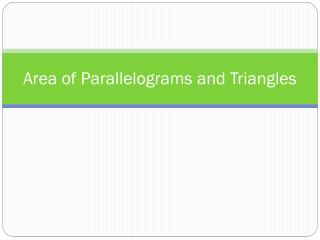DownloadDownload PresentationArea of Parallelograms and Triangles

# Area of Parallelograms and Triangles

Télécharger la présentation## Area of Parallelograms and Triangles

- - - - - - - - - - - - - - - - - - - - - - - - - - - E N D - - - - - - - - - - - - - - - - - - - - - - - - - - -
##### Presentation Transcript

1. Area of Parallelograms and Triangles

2. Parallelograms in Real Life

3. PARALLELOGRAM What is the area of this parallelogram? S=side H=height B=base CUT HERE!

4. PARALLELOGRAM What is the area of this parallelogram? H=height B=base MOVE TO HERE!

5. PARALLELOGRAM It’s the same as the area of this rectangle! H=height B=base

6. PARALLELOGRAM Areaparallelogram = Base x Height (perpendicular height H) Side H=height B=base

7. To find the area of a parallelogram, you always multiply the base and the height. So, Area = base x height or A = bh Remember: The height is the line segment that is perpendicular to the base and creates a right angle. What is the area of this parallelogram? 10 in 12 in Area = base x height A = (10)(10) A = 100 in² 10 in

8. Your Turn • Find the area and perimeter of the parallelogram. A=bh A= 12(24) A=288 cm² 24 cm 14 cm P = 12+12+14+14 P = 52 cm 12 cm

9. Example • Find the area and perimeter of the parallelogram. A=bh A= 15(16) A= 240 cm² 21 cm 16 cm P = 15+15+21+21 P = 72cm 15 cm

10. Triangles in Real Life

11. Area of a Triangle Area of parallelogram = base x height Area of triangle = base x height Area = bh 1 2

12. Finding the Area of a Triangle • Write the formula for area of a triangle. • Substitute in values. • Simplify. A= bh A= (23)(11) A= 126.5 ft² 11 ft 23 ft

13. You Try! • Find the Area of the triangle. • A = ?, b = 9 ft, h = 6 ft A= bh A= (16.1)(12) A= 96.6 m² A= bh A= (9)(6) A= 27 ft² 12 m 16.1 m

14. You try! Find the area and perimeter of the triangle. 20 m A= bh A= (17)(13.4) A= 113.9 m² 15 m P = 20+15+17 P = 52 m 13.4 m 17 m